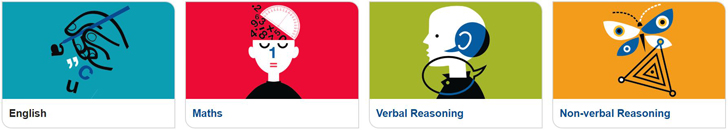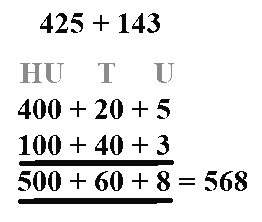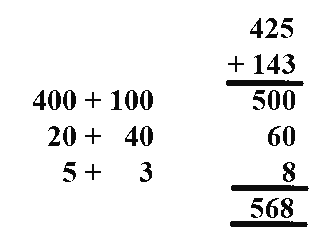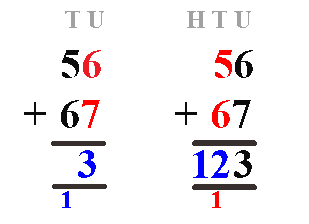## Estimate and Check

Before writing out a calculation it is a good idea to make an estimate first. This is a simple and quick process which helps to ensure that you do not make silly mistakes.

Say you need to add 402 to 97 - simply round the first number 402 to 400, then round the second number 97 to 100 - add the two rounded numbers, 400 + 100 = 500 and you now know that your final answer should be close to 500. When you have completed your calculation, check that it is similar to your estimate and that it makes sense.

## Splitting Method

This method involves partitioning the numbers into 100's, 10's and units, adding these individually and then combining the results to give a final answer.

You can do this by splitting across

#####or by splitting downThis method shows clearly what is happening when two numbers are added together and is great for understanding the process - however it takes time and quite a lot of writing.

## Standard Method

This is the preferred method and requires a good understanding of place value and number bonds up to 20. It is important to keep the numbers in the correct columns (using lined paper sideways, so that the lines are vertical, helps with this).

First add the units - 6 + 7 = 13 , put the 3 in the units column below the question and the 1 (which is a ten) in the tens column.

Next add the tens - 5 + 6 + 1 = 12, place the 2 in the tens column below the question and the 1 (which is a hundred in the hundreds column.)